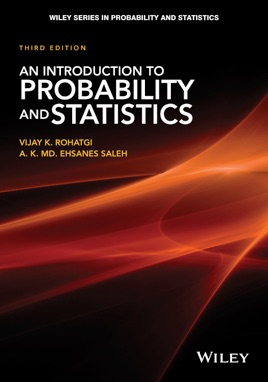• USD 114.99

## Descripción de editorial

A well-balanced introduction to probability theory and mathematical statistics

Featuring updated material, An Introduction to Probability and Statistics, Third Edition remains a solid overview to probability theory and mathematical statistics. Divided intothree parts, the Third Edition begins by presenting the fundamentals and foundationsof probability. The second part addresses statistical inference, and the remainingchapters focus on special topics.

An Introduction to Probability and Statistics, Third Edition includes:
A new section on regression analysis to include multiple regression, logistic regression, and Poisson regression A reorganized chapter on large sample theory to emphasize the growing role of asymptotic statistics Additional topical coverage on bootstrapping, estimation procedures, and resampling Discussions on invariance, ancillary statistics, conjugate prior distributions, and invariant confidence intervals Over 550 problems and answers to most problems, as well as 350 worked out examples and 200 remarks Numerous figures to further illustrate examples and proofs throughout
An Introduction to Probability and Statistics, Third Edition is an ideal reference and resource for scientists and engineers in the fields of statistics, mathematics, physics, industrial management, and engineering. The book is also an excellent text for upper-undergraduate and graduate-level students majoring in probability and statistics.